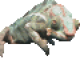# Category Theories: Some definitions to build on

Sorry, but I actually jumped the gun a bit on Yoneda’s lemma.

As I’ve mentioned, one of the things that I don’t like about category theory is how definition-heavy it is. So I’ve been trying to minimize the number of definitions at any time, and show interesting results of using the techniques of category theory as soon as I can.

Well, there are some important definitions which I haven’t talked about yet. And for Yoneda’s lemma to make sense, you really need to see some more examples of how categories express structure. And to be able to show how category theory lets you talk about structure, we need to plough our way through a few more definitions.

### Initial Objects

Suppose we have a category, C. An object o ∈ Obj(C) is called an initial object in C if/f (∀ b ∈ Obj(C)), (∃ 1 f : o → b ∈ Arrows(C)).

In english: an object o is initial in C if there’s exactly one arrow from o to each other object in C. We often write “0C” for the initial object of a category C, or just “0” if it’s obvious what category we’re talking about.

There’s a dual notion of a terminal object: an object is terminal if there’s a exactly one arrow from every object in the category to it. Terminals are written “1C” or just “1”.

Given two objects in a category, if they’re both initial, they must be isomorphic. It’s pretty easy to prove: here’s the sketch. Remember the definition of isomorphism in category theory. An isomorphism is an arrow f : a → b, where (∃ g : b → a) such that f &ormd; g = 1b and g º f = 1a. If an object is initial, then there’s an arrow from it to every other object. Including the other initial object. And there’s an arrow back, because the other one is initial. The iso-arrows between the two initials obviously compose to identities.

### Categorical Products

The product of two morphisms is a generalization of the cartesian product of two sets. It’s important because products are one of the major ways of building complex structures using simple categories.

Given a category C, and two objects a,b ∈ Obj(C), the categorical product a × b consists of:

1. An object p, often written a×b;
2. two arrows pa and pb, where p ∈ Obj(C), pa : p → a, and pb : p → b.
3. a “pairing” operation, which for every object c ∈ C, maps the pair of arrows f : c → a and g : c → b to an arrow Pairc(f,g) : c → a×b. Pairc(f,g) is often written <f,g>c>.

Pairc must have three properties.

1. pa º Pairc(f,g) = f.
2. pb º Pairc(f,g) = g.
3. (∀ h : c → a×b) Pairc(pa º h, pb º h) = h.

The first two of those properties are the separation arrows, to get from the product to its components; and the third is the merging arrow, to get from the components to the product. We can say the same thing about the relationships in the product in an easier way using a commutative diagram:## 0 thoughts on “Category Theories: Some definitions to build on”

1.Daniel Martin

Am I to take this to imply that all categories are closed under categorical product? That seems to me bizarre, at least.
However, the alternative seems to be that one can only define the categorical product of a and b in the presence of a larger category that happens to include an element a×b that fits the definitions above. I suppose that’s ok.

2.Mark C. Chu-Carroll

Daniel:
No, categories are not closed under product. A product of two objects in a category exists only if there is an object and a set of arrows that meet the definition above. If, for two objects in a category, the category doesn’t have the objects and morphisms required by the definition of product, the categorical product of those two objects simply doesn’t exist – the product can’t be taken in the category.
Some categories are closed under product – a special, and extremely interesting family of categories called the cartesian closed categories.

3.elie

i have created a new theory or mathematical formula and actually i dont know where to go and how to authenticate it